# Kelly Criterion – Part 3a – Backing and Laying Bets with Betfair

This post is Part 3 of a series on the Kelly criterion and its application to sports betting. Part 1 provides an introduction to the Kelly criterion along with a worked example. Part 2 provides a simple derivation of the Kelly criterion. Part 3 in this series provides some extensions to the Kelly criterion. This post extends the Kelly criterion to incorporate backing and laying bets using an exchange such as Betfair. It is recommended that you read Part 1 and Part 2 before proceeding, if you have not done so already.

### What is backing and laying?

With standard bookmakers you can only bet for an outcome to occur. Because Betfair is an exchange, rather than a bookmaker, you can bet both for and against sporting outcomes.

If you ‘back’ a bet on an outcome, you are betting that the outcome will happen. For example you could back a bet on Federer to win Wimbledon. If the odds are 3.50, a \$10 bet would result in a \$25 profit if he wins, and a \$10 loss if he doesn’t.

If you ‘lay’ a bet against an outcome, you are betting that the outcome will not happen. For example you could lay a bet on Federer to win Wimbledon. If the odds are 3.50, a \$10 bet would result in a \$25 loss if he wins, and a \$10 profit if Federer doesn’t win. Essentially, when you lay a bet, you act as the bookmaker. You get to keep the other punter’s money if they lose, but have to pay them at the agreed odds if they win.

The Kelly criterion is designed for making ‘back’ bets. According to the formula, you would not make a bet if f < 0. This post adjusts the Kelly criterion to account for Betfair commissions. It also provides the corresponding formula for a lay bet.

### Betfair commissions

Unlike standard bookmakers, who incorporate their fees directly into the odds themselves, Betfair charges a commission fee to the winner of each bet. The commission fee is calculated as a percentage of your net winnings (profit) as follows:

Commission = (market base rate) * (1 – discount rate)

The market base rate is typically 5%. The discount rate ranges between 0% and 60%, depending the level of your account activity. The more bets you make, the greater the discount. A discount rate of 60% corresponds to a commission of 2%, hence the commission fee charged by Betfair ranges between 2% and 5% of your net winnings.

Henceforth we will denote the commission fee (as a percentage of your net winnings) as c.

### Notation

This post continues with the notation used in Part 1 and Part 2. Let:

• W0 = your account balance before you make a bet
• Wn = your account balance after making n bets
• f = the fraction of your bankroll (account balance) to bet on a particular outcome of a sporting event
• d = decimal betting odds for that outcome (2.50 means a winning \$10 back bet would have a payoff of \$25 and a profit of \$15)
• p = your perceived probability of an outcome occurring (where 0 < p < 1)
• q = 1 – p = probability of an outcome not occurring

This post will refer to the hypothetical Arsenal vs Chelsea fixture that was used in Part 1. To recap, the odds are as follows, with your perceived probabilities of occurrence shown in brackets:

Full-time result:

Arsenal: 2.60 (pA = 20% chance of occurring)
Chelsea: 2.65 (pC = 50% chance of occurring)
Draw: 3.25 (pD = 30% chance of occurring)

For simplicity’s sake, it is assumed that due to the high liquidity of the betting market, the lay odds equal the back odds for each outcome. In reality, the lay odds are always slightly above the back odds. The more popular (and liquid) the market, the closer the back and lay odds are to each other.

Example odds in a popular market: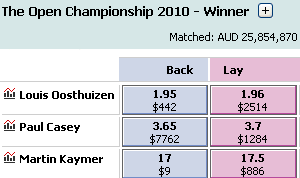Example odds in an unpopular market: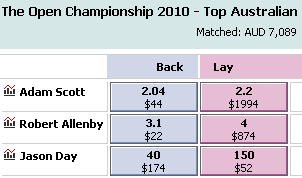### The Kelly criterion for backing a bet

Recall that in Part 2 the exponential rate of growth of the gambler’s capital is expressed as:

(1/n)*log(Wn/W0) = log[(1 + f*(d-1))k(1 – f)(n-k)]1/n

The blue text represents the gross return for a win and the red text represents the gross return for a loss. In Betfair with a commission fee of c, the gross return for winning becomes (1 + f*(d-1)(1-c))k, while the gross return when your bet loses remains unchanged (no commissions are charged to the loser). If you plug this expression into the workings in Part 2 and solve for f, you get: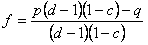The formula above is for the decimal odds system that is popular in Australia. For those who are more familiar with the “b to 1” betting odds used in other literature, the corresponding formula is: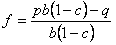Note that for c = 0, the above formulas collapse to the original Kelly criterion used in Part 1 and Part 2 of this series. By taking the derivative of the expression for f with respect to c you will find that as c increases, f decreases. This is because the expected payoff for the bet drops as the commission rises, which makes the bet less worthwhile. Recall the example from Part 1:

Arsenal: fA = [0.2(2.6 – 1) – 0.80]/(2.6 – 1) = –0.300
Chelsea: fC = [0.5(2.65 – 1) – 0.50]/(2.65 – 1) = 0.197
Draw: fD = [0.3(3.25 – 1) – 0.70]/(3.25 – 1) = –0.011

If these odds were offered by Betfair, we need to adjust them for the commission fee using the formula presented above. For a commission fee of 5% the figures become:

Arsenal: fA = [0.2(2.6 – 1)(1 – 0.05) – 0.80]/((2.6 – 1)(1 – 0.05)) = –0.326
Chelsea: fC = [0.5(2.65 – 1)(1 – 0.05) – 0.50]/((2.65 – 1)(1 – 0.05) = 0.181
Draw: fD = [0.3(3.25 – 1)(1 – 0.05) – 0.70]/((3.25 – 1)(1 – 0.05) = –0.027

Now you would bet 18.1% of your account balance on Chelsea instead of 19.7%.

### The Kelly criterion for laying a bet

When you lay a bet in Betfair you receive the other punter’s bet as a payout if the outcome does not occur, but you have to pay the punter at the agreed odds if the outcome does occur. As before, let p denote your perceived probability of the outcome occurring. In this case, you will lose the bet if the outcome does occur, and win the bet if it doesn’t.

Your account balance if you win will be W0(1 + f*(1-c)), while your account balance if you lose will be W0(1 – f*(d-1)).

Note that we must place the following restriction on f: 0 < f*(d-1) < 1, or equivalently, 0 < f < 1/(d-1). This prevents losing an amount that is greater than your account balance. You can think of this as your budget constraint. This contrasts with the budget constraint for backing an outcome: 0 < f < 1. Note that for a low odds bet of 1.01 and an account balance of \$100, you could lay a bet amount up to \$10,000, which is more than your account balance. This is because your total potential liability is (\$10,000)(1.01 - 1) = \$100. In contrast, for high odds of 101.00, the maximum you could lay for this bet is \$1, which is far lower than your account balance of \$100. We use < rather than < for the upper limit to avoid the possibility of your account dropping to zero.

After n bets, k of which result in the outcome occurring (a loss for you), and (n-k) of which result in the outcome not occurring (a win for you), your account balance will be:

Wn = W0(1 – f*(d-1))k(1 + f*(1-c))(n-k)

We can express the exponential rate of growth of the gambler’s capital as:

G = (1/n)*log(Wn/W0) = log[(1 – f*(d-1))k(1 + f*(1-c))(n-k)]1/n

Setting the derivative of dG/df equal to zero and solving for f gives:The formula above is for the decimal odds system. For those who are more familiar with the “b to 1” betting odds used in other literature, the corresponding formula is: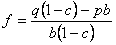If the above workings are difficult to follow, I recommend re-reading Part 2 of this series.

Referring back to the Arsenal vs Chelsea example, in the absence of commission fees you would calculate f as :

Arsenal: fA = [0.8 – 0.2*(2.6 – 1)]/(2.6 – 1) = 0.300
Chelsea: fC = [0.5 – 0.5*(2.65 – 1)]/(2.65 – 1) = –0.197
Draw: fD = [0.7 – 0.3*(3.25 – 1)]/(3.25 – 1) = 0.011

Note that these values are the exact opposites in sign of the corresponding figures for back bets in the absence of commission fees. For a commission fee of 5%, you would calculate f as:

Arsenal: fA = [0.8(1 – 0.05) 0.2*(2.6 – 1)]/[(2.6 – 1)(1 – 0.05)] = 0.289
Chelsea: fC = [0.5(1 – 0.05) – 0.5*(2.65 – 1)]/[(2.65 – 1)(1 – 0.05)] = –0.223
Draw: fD = [0.7(1 – 0.05) – 0.3*(3.25 – 1)]/[(3.25 – 1)(1 – 0.05)] = –0.005

Based on these figures you would choose to lay a bet against Arsenal using 28.9% of your account balance. It should be immediately noted that in doing so, it isn’t 28.9% of your balance that is at risk. If Arsenal fails to win, you would receive a profit equal to 28.9%(1 – 0.05) = 27.7455% of your account balance. If Arsenal does win, you will lose (2.60 – 1)(.289) = 46.24% of your account balance. Hence, when making a lay a bet, the fraction (d-1)f of your account balance is at risk and unavailable to bet elsewhere.

### Should I back a bet or lay a bet?

In the example fixture between Arsenal and Chelsea, based on the Kelly criterion, you could back Chelsea using 18.1% of your account balance, or you could lay a bet against Arsenal using 28.9% of your account balance. So which action should you take? Should you do both?

Recall that the objective in Kelly betting is to maximise the exponential growth rate of your account balance. Let’s compare the expected exponential growth rate for:

A: Making a back bet on Chelsea
B: Making a lay bet against Arsenal
C: Making a back bet on Chelsea and making a lay bet against Arsenal

In formula form, your account balance for each strategy after each possible outcome is provided below.

 OutcomeArsenal WinChelsea WinDraw ProbabilitypApCpD Option A PayoffW0(1 – fBC)W0[1 + (1-c)(dC-1)fBC]W0(1 – fBC) Option B PayoffW0[1 – (dA-1)fLA]W0[1 + (1-c)fLA]W0[1 + (1-c)fLA] Option C PayoffW0[1 – fBC – (dA-1)fLA]W0[1 + (1-c)(dC-1)fBC + (1-c)fLA]W0[1 – fBC + (1-c)fLA]

Plugging in the values for our Arsenal vs Chelsea example gives:

 OutcomeArsenal WinChelsea WinDraw Probability0.20.50.3 Option A PayoffW0(1 – 0.181)W0[1 + (0.95)(1.65)(0.181)]W0(1 – 0.181) Option B PayoffW0[1 – (1.6)(0.289)]W0[1 + (0.95)(0.289)]W0[1 + (0.95)(0.289)] Option C PayoffW0[1 – 0.181 – (1.6)(0.289)]W0[1 + (0.95)(1.65)(0.181) + (0.95)(0.289)]W0[1 – 0.181 + (0.95)(0.289)]

Note that the numbers below will differ slightly to the values calculated using the rounded figures above. Solving gives:

 OutcomeArsenal WinChelsea WinDraw Probability0.20.50.3 Option A PayoffW0(0.8190)W0(1.2838)W0(0.8190) Option B PayoffW0(0.5368)W0(1.2750)W0(1.2750) Option C PayoffW0(0.3558)W0(1.5588)W0(1.0940)

Let vi denote the profit/loss for outcome i. A win will be associated with vi > 0 and a loss will be associated with vi < 0. We can calculate the expected exponential growth rate for each strategy using the formula:

W1/W0 = pA*log(1 + vA) + pC*log(1 + vC) + pD*log(1 + vD)

Option A: W1/W0 = 0.2*log(0.8190) + 0.5*log(1.2838) + 0.3*log(0.8190) = 0.011
Option B: W1/W0 = 0.2*log(0.5368) + 0.5*log(1.2750) + 0.3*log(1.2750) = 0.030
Option C: W1/W0 = 0.2*log(0.3558) + 0.5*log(1.5588) + 0.3*log(1.0940) = 0.018

In this example, option B of laying a bet against Arsenal provides the greatest expected exponential growth rate of the account balance. Hence based on the Kelly criterion and the above perceived probabilities of occurrence, you should make the single bet of laying 28.9% of your account balance against Arsenal to win.

### Coming up in Part 3b

The next post will provide an extension to the Kelly criterion to account for the time value of money. Time is an important consideration when the bet settlement is in the distant future.

## 7 Responses to "Kelly Criterion – Part 3a – Backing and Laying Bets with Betfair"

1.Situs Judi Baccarat

Good write-up. I definitely appreciate this site. Keep writing!

2.livebid

Greetings! The very helpful advice in this particular post related to back lay betting

3.Nice post it is very much informative love to read this about back lay betting ,as it will be very much helpful for players who are intrested in sports betting tips.

This site uses Akismet to reduce spam. Learn how your comment data is processed.